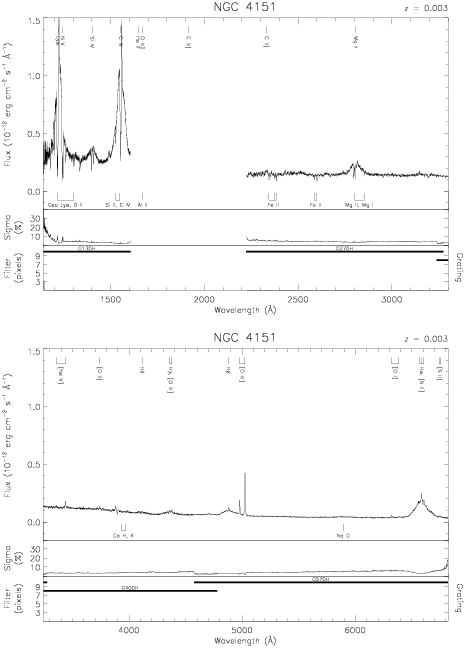Download dataset
TELESCOP= 'HST     '
INSTRUME= 'FOS     '
EQUINOX =               2000.0
DATE-OBS= '1992-06-22T02:25:26'
RA_PNT  =       182.6354982219
DEC_PNT =        39.4057774947
PA_PNT  =       276.7701503185
OBJECT  = 'NGC 4151'
ROOTNM01= 'Y0YC0102T'
GRNDMD01= 'SPECTROSCOPY'
DETECT01= 'BLUE    '
APERID01= 'B-3     '
FGWAID01= 'H13     '
ROOTNM02= 'Y0YC0103T'
GRNDMD02= 'SPECTROSCOPY'
DETECT02= 'BLUE    '
APERID02= 'B-3     '
FGWAID02= 'H13     '
ROOTNM03= 'Y0YC0104T'
GRNDMD03= 'SPECTROSCOPY'
DETECT03= 'BLUE    '
APERID03= 'B-3     '
FGWAID03= 'H13     '
ROOTNM04= 'Y0YC0202T'
GRNDMD04= 'SPECTROSCOPY'
DETECT04= 'BLUE    '
APERID04= 'B-3     '
FGWAID04= 'H13     '
ROOTNM05= 'Y0YC0203T'
GRNDMD05= 'SPECTROSCOPY'
DETECT05= 'BLUE    '
APERID05= 'B-3     '
FGWAID05= 'H13     '
ROOTNM06= 'Y0YC0204T'
GRNDMD06= 'SPECTROSCOPY'
DETECT06= 'BLUE    '
APERID06= 'B-3     '
FGWAID06= 'H13     '
ROOTNM07= 'Y0YC0402T'
GRNDMD07= 'SPECTROSCOPY'
DETECT07= 'BLUE    '
APERID07= 'B-3     '
FGWAID07= 'H13     '
ROOTNM08= 'Y0YC0403T'
GRNDMD08= 'SPECTROSCOPY'
DETECT08= 'BLUE    '
APERID08= 'B-3     '
FGWAID08= 'H13     '
ROOTNM09= 'Y0YC0404T'
GRNDMD09= 'SPECTROSCOPY'
DETECT09= 'BLUE    '
APERID09= 'B-3     '
FGWAID09= 'H13     '
ROOTNM10= 'Y0YC0602T'
GRNDMD10= 'SPECTROSCOPY'
DETECT10= 'BLUE    '
APERID10= 'B-3     '
FGWAID10= 'H13     '
ROOTNM11= 'Y0YC0603T'
GRNDMD11= 'SPECTROSCOPY'
DETECT11= 'BLUE    '
APERID11= 'B-3     '
FGWAID11= 'H13     '
ROOTNM12= 'Y0YC0604T'
GRNDMD12= 'SPECTROSCOPY'
DETECT12= 'BLUE    '
APERID12= 'B-3     '
FGWAID12= 'H13     '
ROOTNM13= 'Y19G0303T'
GRNDMD13= 'SPECTROSCOPY'
DETECT13= 'AMBER   '
APERID13= 'B-2     '
FGWAID13= 'H57     '
ROOTNM14= 'Y19G0304T'
GRNDMD14= 'SPECTROSCOPY'
DETECT14= 'AMBER   '
APERID14= 'B-2     '
FGWAID14= 'H40     '
ROOTNM15= 'Y19G0305T'
GRNDMD15= 'SPECTROSCOPY'
DETECT15= 'AMBER   '
APERID15= 'B-2     '
FGWAID15= 'H27     '